• 特征点提取与匹配
千次阅读
2018-09-09 15:35:36

# 特征点提取与匹配

## 特征点概述

• 如何高效且准确的匹配出两个不同视角的图像中的同一个物体，是许多计算机视觉应用中的第一步。虽然图像在计算机中是以灰度矩阵的形式存在的，但是利用图像的灰度并不能准确的找出两幅图像中的同一个物体。这是由于灰度受光照的影响，并且当图像视角变化后，同一个物体的灰度值也会跟着变化。所以，就需要找出一种能够在相机进行移动和旋转（视角发生变化），仍然能够保持不变的特征，利用这些不变的特征来找出不同视角的图像中的同一个物体。

• 为了能够更好的进行图像匹配，需要在图像中选择具有代表性的区域，例如：图像中的角点、边缘和一些区块，但在图像识别出角点是最容易，也就是说角点的辨识度是最高的。所以，在很多的计算机视觉处理中，都是提取交掉作为特征，对图像进行匹配，例如SFM，视觉SLAM等。

• 例如：相机从远处得到的是角点，但是在近处就可能不是角点；或者，当相机旋转后，角点就发生了变化。为此，计算机视觉的研究者们设计了许多更为稳定的的特征点，这些特征点不会随着相机的移动，旋转或者光照的变化而变化。例如：SIFT,SURF,ORB等

• 一个图像的特征点由两部分构成：关键点（Keypoint）和描述子（Descriptor）。

关键点指的是该特征点在图像中的位置，有些还具有方向、尺度信息；描述子通常是一个向量，按照人为的设计的方式，描述关键点周围像素的信息。通常描述子是按照外观相似的特征应该有相似的描述子设计的。因此，在匹配的时候，只要两个特征点的描述子在向量空间的距离相近，就可以认为它们是同一个特征点。

• 特征点的匹配通常需要以下三个步骤

• 提取图像中的关键点，这部分是查找图像中具有某些特征（不同的算法有不同的）的像素
• 根据得到的关键点位置，计算特征点的描述子
• 根据特征点的描述子，进行匹配

### 特征点描述子

• 一个好的描述子是准确匹配的基础
• 从图像中提取到特征的关键点信息，通常只是其在图像的位置信息（有可能包含尺度和方向信息），仅仅利用这些信息无法很好的进行特征点的匹配 ，所以就需要更详细的信息，将特征区分开来，这就是特征描述子
• 特征的描述子通常是一个精心设计的向量，描述了关键点及其周围像素的信息。
• 为了能够更好的匹配，一个好的描述子通常要具有以下特性：
• 不变性 指特征不会随着图像的放大缩小旋转而改变。
• 鲁棒性 对噪声、光照或者其他一些小的形变不敏感
• 可区分性 每一个特征描述子都是独特的，具有排他性，尽可能减少彼此间的相似性。
• 特征点的不变性主要体现在两个方面:
• 尺度不变性
• 旋转不变性

### 常用的特征点算法

• 图像的特征点包含两个部分
• 特征点的提取，在图像检测到特征点的位置
• 特征点的描述，也就是描述子。

## OpenCV3中的特征图提取与匹配



int main() {

// 1.初始化
std::cout << "init" << std::endl;
vector<KeyPoint> keypoints1, keypoint2;
Mat descriptors1, descriptors2;

Ptr<ORB> orb = ORB::create();

// 2.提取特征点
std::cout << "detect" << std::endl;
orb->detect(img1, keypoints1);
orb->detect(img2, keypoint2);

// 3.计算特征描述符
std::cout << "compute" << std::endl;
orb->compute(img1, keypoints1, descriptors1);
orb->compute(img2, keypoint2, descriptors2);

// 4.对两幅图像的BRIEF描述符进行匹配,使用BFMatch,Hanming距离作为参考.
std::cout << "match" << std::endl;
vector<DMatch> matches;
BFMatcher bfMatcher(NORM_HAMMING);
bfMatcher.match(descriptors1, descriptors2, matches);

//imshow("output", matches);

std::cout << "draw matches" << std::endl;
Mat image_matches;
drawMatches(img1, keypoints1, img2, keypoint2, matches,
image_matches, Scalar(0, 255, 0), Scalar::all(-1), vector<char>(), 0);

imshow("matches", image_matches);

waitKey(0);
return 0;
}
更多相关内容
• surf算法在opencv3上实现，自己实现代码不用调用任何包。
• 针对将彩色图像转化为灰度图像后再进行特征点提取与匹配会丢失彩色信息，可能导致误匹配这一问题，采用一种基于彩色信息的SIFT特征点提取匹配算法（CSIFT）以实现彩色图像的特征点提取匹配，结合目标的颜色特征...
• 一个cpp文件。 功能：利用opencv库对SIFT角进行提取，并进行匹配，最后画出匹配示意图。程序逻辑简单，可以在内部自由改动。
• ## 图像配准，特征点提取与匹配

万次阅读 多人点赞 2019-03-26 12:20:55
图像配准 本文为原创文章，转载请注明...匹配：指寻找两幅影像中相似的部分(基于特征点或灰度等)，从而找到搜索图像相似的图像 配准：将不同时间、不同传感器(成像设备)或不同条件下(天候、照度、摄像位置和角度...

# 图像配准

本文为原创文章，转载请注明出处**

## 目录

一、图像配准概述
二、图像匹配算法
SIFT
SURF
ORB
暴力匹配
FLANN
深度学习（superpoint）
三、各算法对比
四、图像配准在SLAM中的应用

## 图像配准概述

匹配：指寻找两幅影像中相似的部分(基于特征点或灰度等)，从而找到与搜索图像相似的图像
配准：将不同时间、不同传感器(成像设备)或不同条件下(天候、照度、摄像位置和角度等)获取的两幅或多幅图像进行匹配、叠加的过程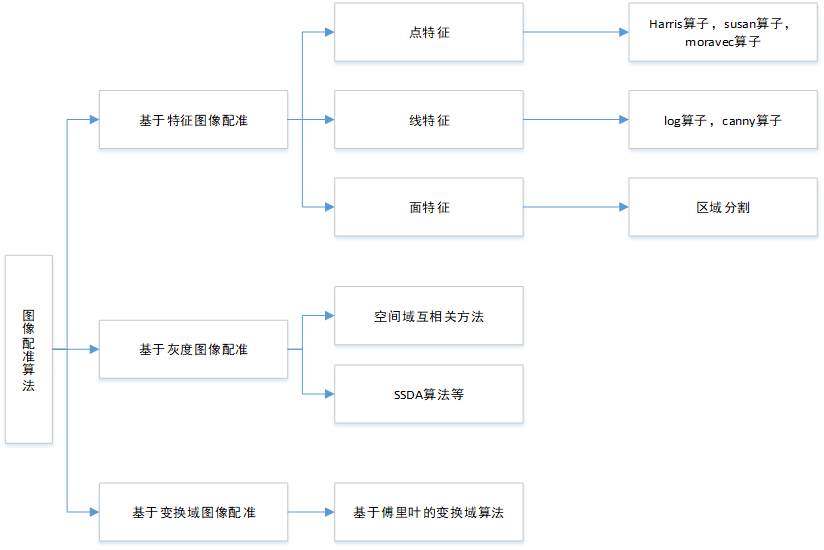### 特征点

特征点：图像当中具有代表性的部分
可重复性：相同的区域可在不同的图像中被找到。
可区别性：不同的区域有不同表达。
高效率：同一图像中，特征点的数量应远小于像素的数量。
本地性：特征仅与一小片图像区域有关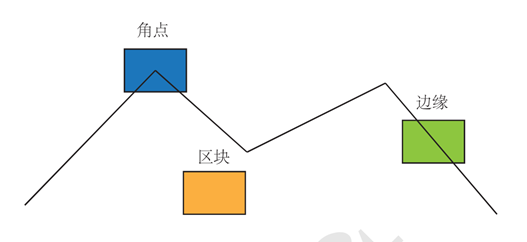特征点信息
位置、大小、方向、评分等------关键点
特征点周围图像的信息------描述子

特征描述应该在光照、视角发生少量变化时仍能保持一致

### 基于特征点配准的优缺点

■ 提取待配准图像中的点、线、边缘等特征信息，不需要其它辅助信息，在减少计算量、提高效率的同时，能够对图像灰度的变化有一定的鲁棒性
■ 只采用了图像一小部分的特征信息，所以这种算法对特征提取和特征匹配的精度及准确性要求非常高，对错误非常敏感
■ 特征点所包含的信息相对较少，只能反映出其在图像中的位置坐标信息，所以在两幅图像中寻找匹配的特征点是关键所在

### 基于特征图像的配准流程

1.首先对两幅图像进行特征提取得到特征点；
2.通过进行相似性度量找到匹配的特征点对；
3.然后通过匹配的特征点对得到图像空间坐标变换参数；
4.最后由坐标变换参数进行图像配准。

### 高斯金字塔与尺度空间

■ 高斯核是唯一的线性核，对图像模糊不会引入其他噪声
■ 对每层最模糊的一幅图像继续下采样，继续高斯模糊处理
■ 上采样（分辨率逐级升高）和下采样（分辨率逐级降低）
■ 尺度空间（O,S）
– O—octave
– S-层
– （o，s）能够确定高斯金字塔中的唯一一幅图像δ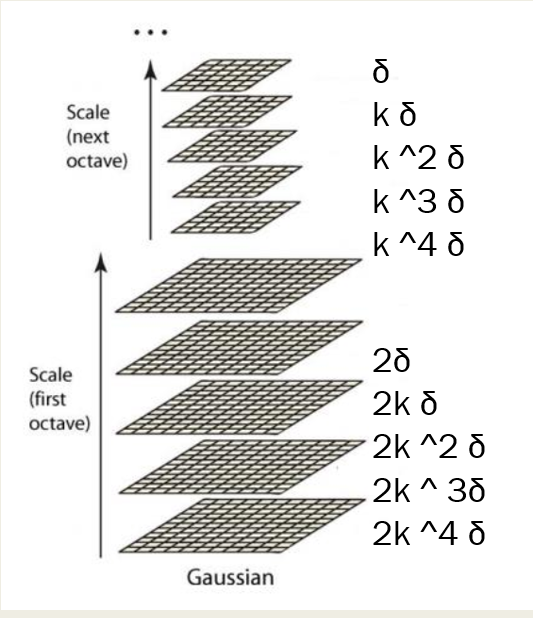其中δ为尺度大小，k的几次幂为每个八度（octave）的第几个模糊层

#### 尺度空间图例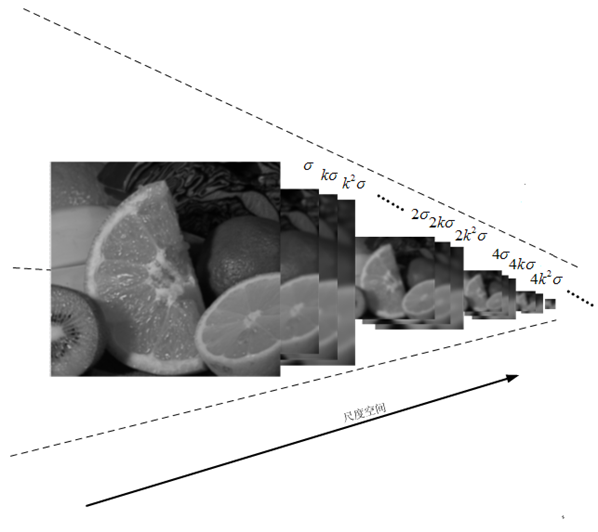#### 差分高斯金字塔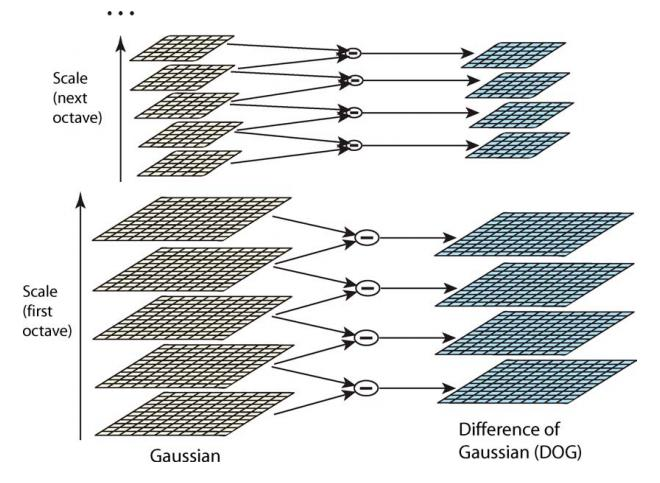同一个八度的相邻两模糊层做差得到差分高斯金字塔

### 特征点检测与描述------SIFT

在不同的尺度空间上查找关键点（特征点），计算关键点的大小、方向、尺度信息，利用这些信息组成关键点对特征点进行描述
具体步骤：
■ 1. 生成高斯差分金字塔（DOG金字塔），尺度空间构建
■ 2. 空间极值点检测 （在高斯金字塔中搜索对尺度和旋转不变的特征点）
■ 3. 稳定关键点的精确定位 （离散空间用曲线拟合寻找极值点）
■ 4. 稳定关键点方向信息分配 （图像局部的梯度方向）
■ 5. 关键点描述 （将得到的特征点位置、方向、尺度信息，使用一组向量来描述特征点及其周围邻域像素的信息）
■ 6. 特征点匹配

#### 优缺点

■ 1. 对旋转、尺度缩放、亮度变化保持不变性，对视角变化、噪声等也存在一定程度的稳定性；
■ 2. 独特性，信息量丰富，适用于在海量特征数据中进行快速，准确的匹配；
■ 3. 多量性，即使少数几个物体也可以产生大量的Sift特征向量；
■ 4. 可扩展性，可以很方便的与其他形式的特征向量进行联合；

#### SIFT结合FLANN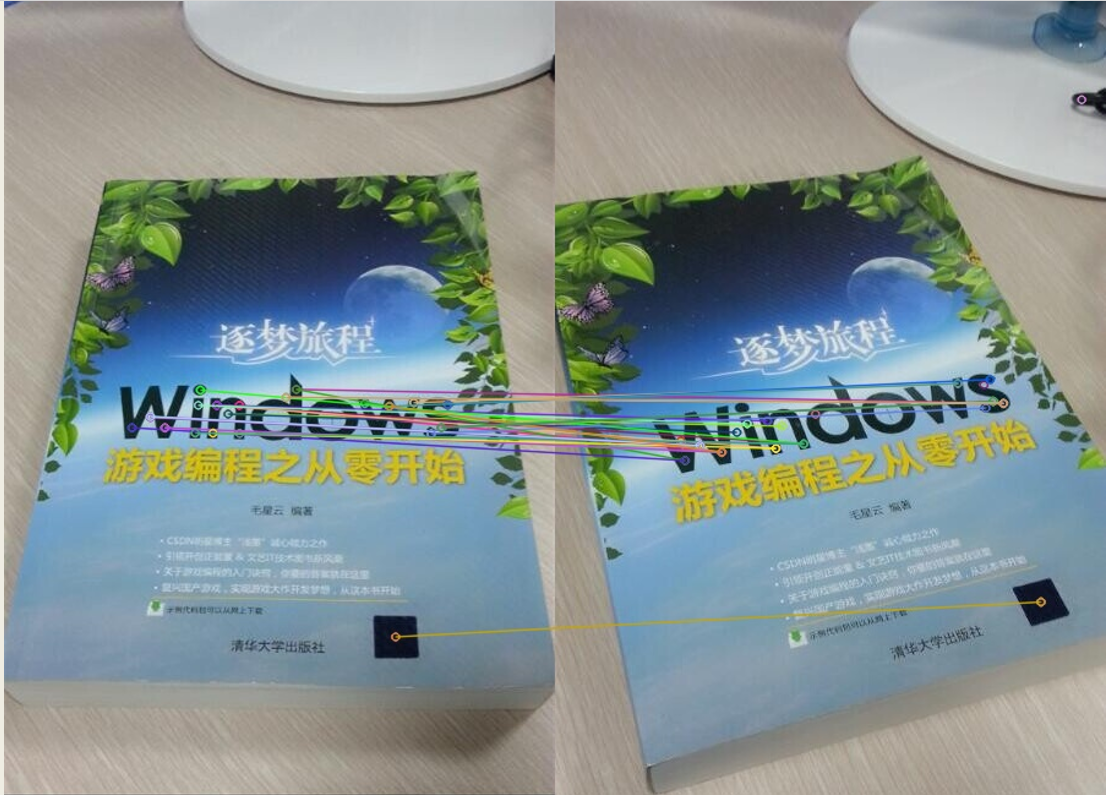### 特征点检测–SURF

■ 1. 用Hessian矩阵构造高斯金字塔尺度空间构建
（高斯滤波后进行矩阵计算构建金字塔）
■ 2. 非极大值抑制初步确定特征点
■ 3. 稳定关键点的精确定位 （连续空间到离散空间）
■ 4. 选取主方向 （统计特征点邻域内的harr小波特征）
■ 5. 关键点描述
16*4=64维
■ 6. 特征点匹配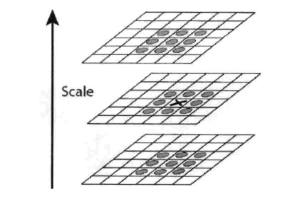通过LOWE的论文可知，SIFT和SURF的速度相差一个数量级
原因
1.描述子的维度不同
SIFT是取1616的邻域分成44的块区域，每块统计八个方向梯度，所以为478=128维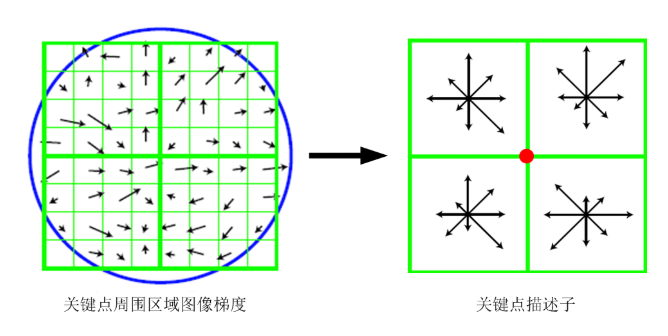SURF是分成16块，每块统计25个点4个方向的小波特征，所以为16*4=64维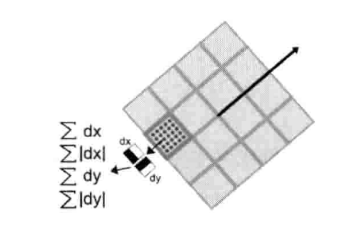2.SIFT是固定高斯核，改变图像尺寸； SURF固定尺寸，改变高斯核，所以SURF可以通过同一尺寸的图像与多个高斯核卷积并行处理

#### 特征检测–SURF效果图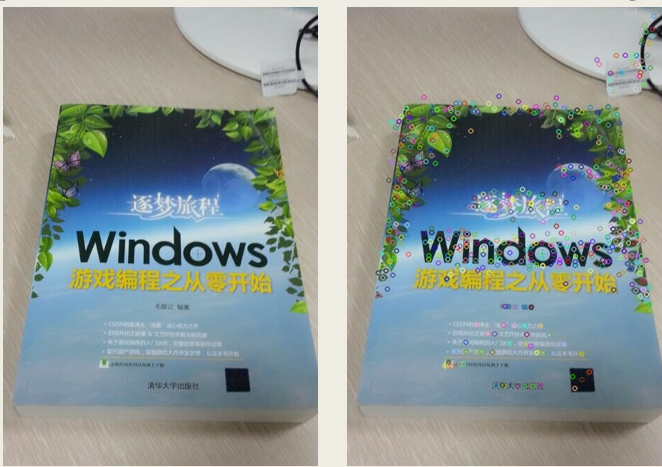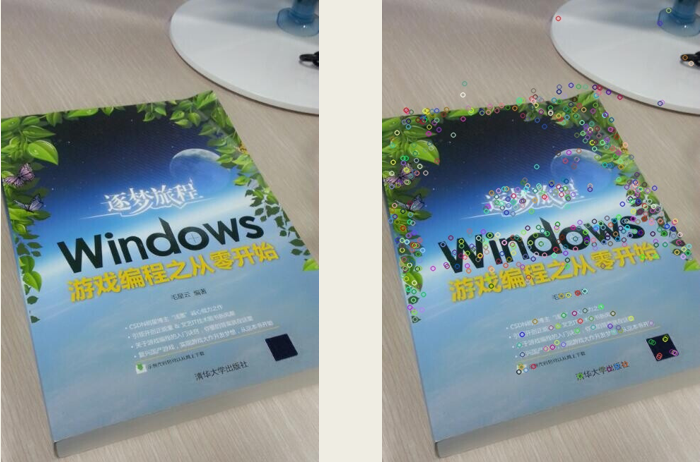#### 特征描述与匹配------SURF效果图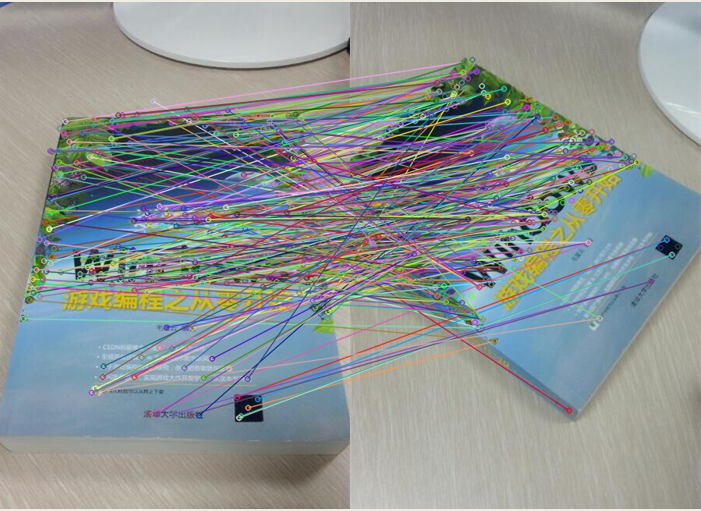上述图片存在误匹配点，可以使用LM算法进行优化同时采用RANSAC算法筛选出内点

### 特征检测------ORB

ORB特征
关键点：oriented FAST
描述： BRIEF

FAST:连续N个点的灰度有明显差异
若某像素与其周围邻域内足够多的像素点相差较大，则该像素可能是角点
如下面介绍FAST-10（连续十个点超过阈值）算法步骤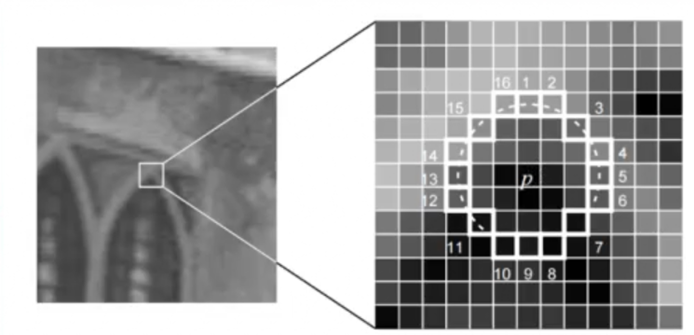算法步骤：
上图所示，
1.一个以像素p为中心，半径为3的圆上，有16个像素点（p1、p2、…、p16）。

2、定义一个阈值。计算p1、p9、p5、p13与中心p的像素差，若它们的绝对值有至少3个超过阈值，则当做候选角点，再进行下一步考察；否则，不可能是角点；

3、若p是候选点，则计算p1到p16这16个点与中心p的像素差，若它们有至少连续10（所以为FAST-10）个超过阈值，则是角点；否则，不可能是角点。

4、对图像进行非极大值抑制：计算特征点出的FAST得分值（即score值，也即s值），判断以特征点p为中心的一个邻域（如3x3或5x5）内，计算若有多个特征点，则判断每个特征点的s值（16个点与中心差值的绝对值总和），若p是邻域所有特征点中响应值最大的，则保留；否则，抑制。若邻域内只有一个特征点（角点），则保留。得分计算公式如下（公式中用V表示得分，t表示阈值）：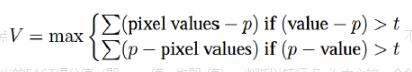FAST算法实现起来简单，尤其是以速度快著称。

oriented Fast：在FAST基础上计算旋转

Brief(binary robust independent elementary features)描述子:
在特征点附近随机选取若干点对，将所选取的点的灰度值组成一个二进制串作为特征点描述子，用汉明距离度量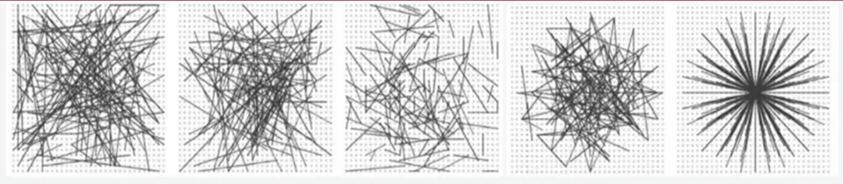速度快，但是不具备旋转不变性和尺度不变性，对噪声敏感

#### 特征检测–ORB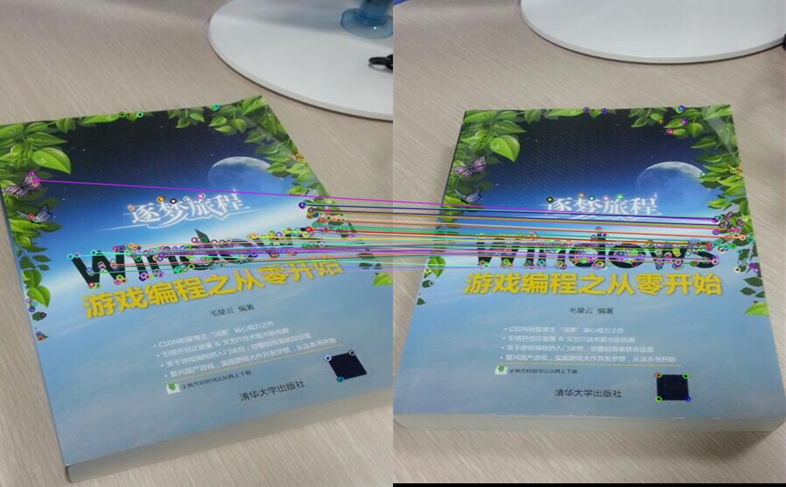### 图像匹配------暴力匹配和FLANN匹配器

图像匹配：通过描述子的差异判断哪些特征为同一点
暴力匹配：比较图A的每个特征的与图B的所有特征点的距离，相当于轮询
加速：快速最近邻（FLANN）

对大数据集和高维特征进行最近邻搜索的算法的集合，如k近邻算法等

暴力匹配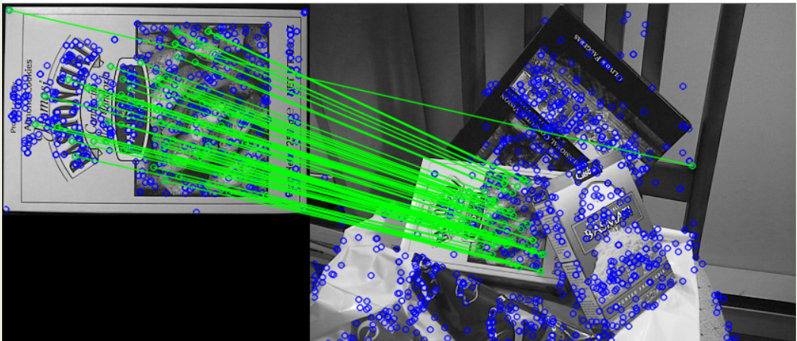### FLANN特征点匹配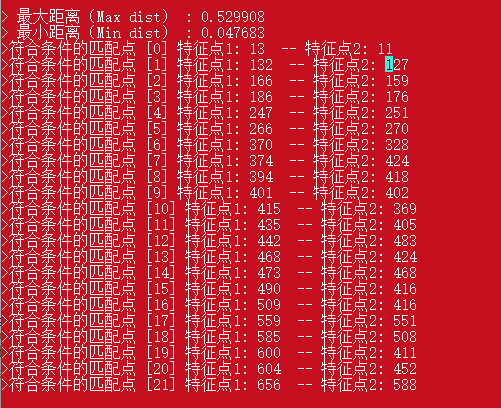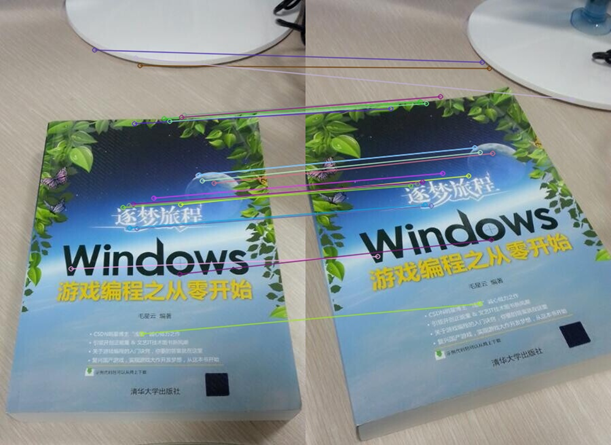用快速近邻方法找到特征点匹配对，速度快，如图中找到了22对匹配的特征点

### SURF和ORB的对比SURF：用时0.6938s，用RANSAC算法和LM算法优化筛选出的匹配点对为243对ORB：用时0.1184s，用RANSAC算法和LM算法优化筛选出的匹配点对为80对

正如论文中所提到的，ORB比SURF快一个数量级，SURF比SIFT快一个数量级

### Self-Supervised Interest Point Detection and Description（基于深度学习）

■ 描述符具有优良特性，在定位场景下，可以对季节和环境光照具有更强的鲁棒性
■ 虽然深度学习目前还不能很好的支持SLAM中的几何计算和位姿估计，但是在特征点跟踪和匹配上可以做得很好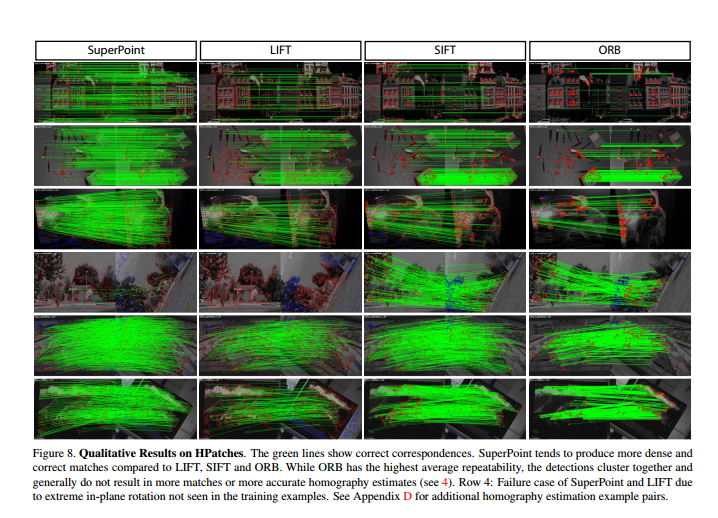## SLAM中的图像配准

实时性必须强，速度必须要快，可以在质量和速度之间折中

■ SIFT,SURF等特征提取方法具有较好的并行性，可通过GPU等设备加速运算，如加速后的SIFT就满足实时性的要求。
■ 在目前的SLAM方案中，ORB是质量与性能之间较好的折中
■ ORB在平移、旋转、缩放的变换下仍具有较好的表现
■ FAST和BRIEF的组合非常高效
■ 匹配的时候采用快速近似最近邻

展开全文SIFT SURF ORB
• matlab源代码，实现特征点匹配与实现，实测有效！前期数据分析验证非常高效，实测有用！实测有用！实测有用！
• 上篇博文中,小楼给大家介绍了SIFT特征点,这中特征点的优势固然明显,但随着带来的副作用也是巨大的.(老天爷从来都是公平的)....它采用关键和二进制描述子来对特征点进行判定描述.下面为大家详细介绍ORB特征. 1. ...

上篇博文中,小楼给大家介绍了SIFT特征点,这中特征点的优势固然明显,但随着带来的副作用也是巨大的.(老天爷从来都是公平的).那就是它巨大的计算量.目前来说,还没有那种cpu能够实时的计算SIFT特征点.
今天,介绍给大家一种相对来说更加完美的特征点–ORB特征.
ORB特征是近年来非常具有代表性的一种特征.它采用关键点和二进制描述子来对特征点进行判定与描述.下面为大家详细介绍ORB特征.
1. ORB特征由FAST关键点和BRIEF描述子构成,
其特征点的提取步骤分为FAST关键点的提取和BRIEF描述子的生成.
2.FAST关键点的提取:
FAST是一种角点,主要根据该点像素值与周围邻域像素点的像素值比较获得.主要检测灰度变化明显的地方.其提取步骤可以分为四部.
(1)读取检测点的灰度值I;
(2)设置一个阈值P(如I的120%);
(3)选取检测点的邻域像素;
(4)判断邻域像素点的灰度值是否大于阈值P,若连续n个邻域点的灰度值大于阈值,判定检测点为关键点 .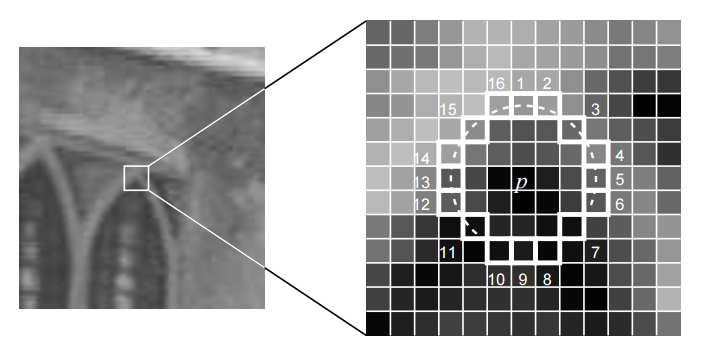BRIEF描述子:BRIEF描述子为一种二进制描述子,通过比较关键点与其邻域点像素值的大小.如邻域点像素值大于关键点像素值的话取0,否则取1.这样我们可以构建出一个128维的二进制向量来对关键点进行描述.就是因为ORB特征的描述子只需要比较大小,因此,它能保持很好的实时性.

接下来是ORB特征的提取实践,编写一个简单的小程序就可以实现这个功能.
运行环境:ubuntu16.04+opencv-3.1.0

 //初始化
std::vector<KeyPoint> keypoints_1;
Mat descriptors_1;

//计时

clock_t time_stt=clock();
cout<<"< Extracting keypoints from images using ORB..."<<endl;
Ptr<FeatureDetector> detector = ORB::create();
detector->detect ( image,keypoints_1 );

Ptr<DescriptorExtractor> descriptor = ORB::create();
descriptor->compute ( image, keypoints_1, descriptors_1 );
cout<<"image has"<<keypoints_1.size()<<"points"<<endl;
cout<<"time use in ORB is"<<1000 * (clock() - time_stt)/(double)CLOCKS_PER_SEC << "ms" << endl;

//绘制并保存特征点
Mat out1;
namedWindow("提取结果",CV_WINDOW_NORMAL);
drawKeypoints( image, keypoints_1, out1, Scalar::all(-1), DrawMatchesFlags::DRAW_RICH_KEYPOINTS);
imshow("提取结果",out1);
imwrite("./orb.png",out1);


提取结果如下图所示: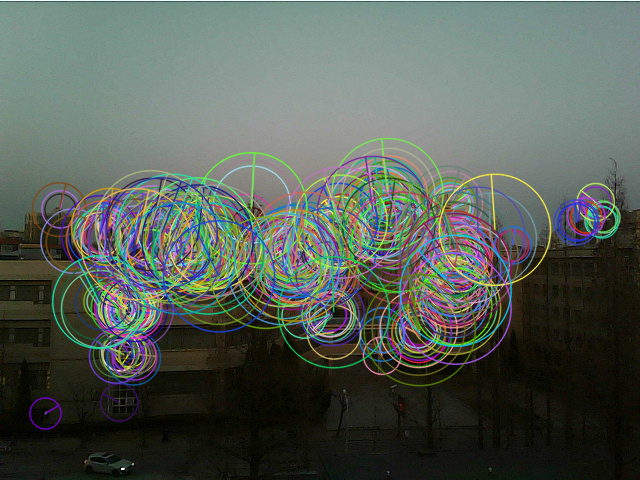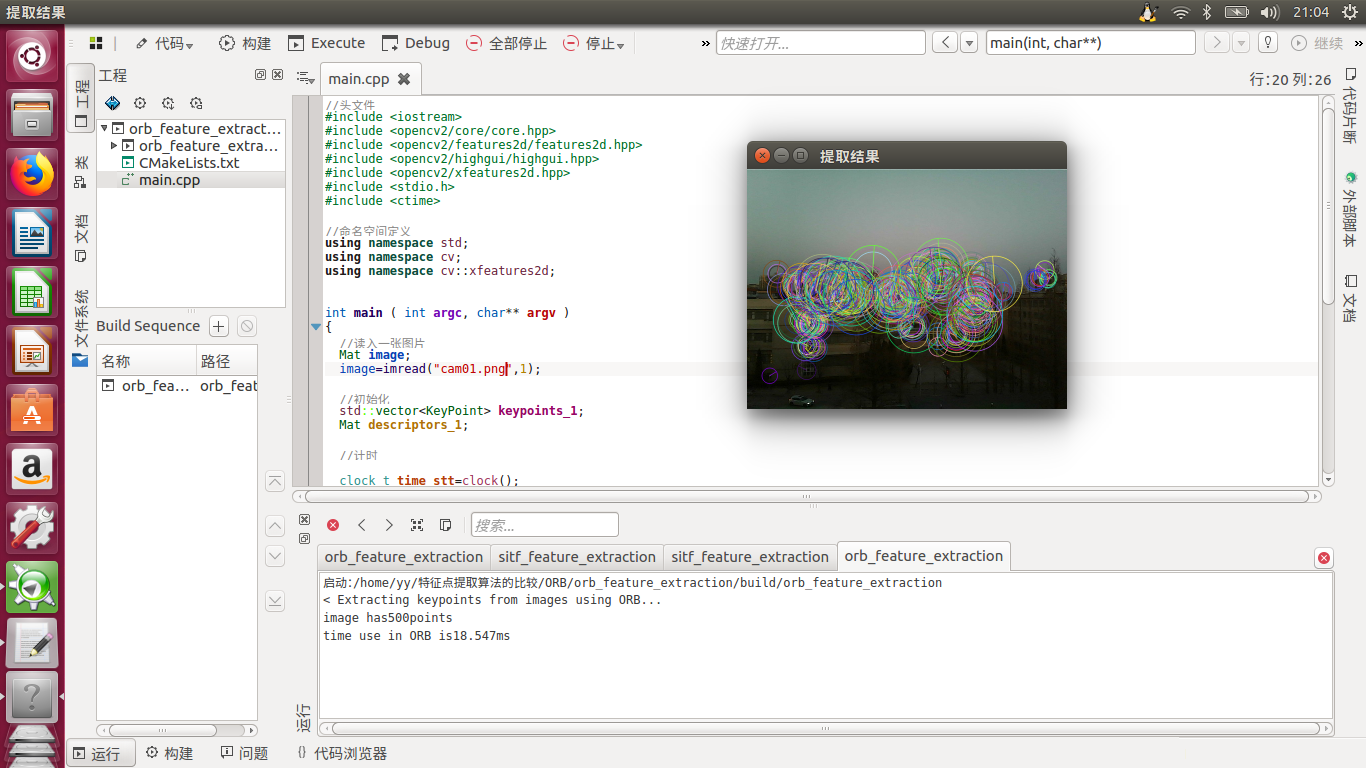大家可以与上文的SIFT特征点进行对比,会发现提取时间大大减少了.这个时间也与电脑的性能有关,但是楼主用的是同一台老电脑.ORB特征的强大可见一斑!

全部代码留邮箱找楼主要,欢迎大家随时讨论!

展开全文• 使用OPENCV实现SIFT算法，完成特征点提取匹配，程序可正常运行
• Opencv 各种特征点提取匹配 class KeyPoint { Point2f pt; //坐标 float size; //特征点邻域直径 float angle; //特征点的方向，值为[零,三百六十)，负值表示不使用 float response; int octave; //特征点...Opencv
• 本文实例为大家分享了利用opencv实现SIFT特征提取与匹配的具体代码，供大家参考，具体内容如下 1、SIFT 1.1、sift的定义 SIFT，即尺度不变特征变换（Scale-invariant feature transform，SIFT），是用于图像处理领域...
• 建立在Moravec算子基础上提取特征点另外一幅图像进行匹配计算，并输出特征点对应的匹配点像素坐标 建立在Moravec算子基础上提取特征点另外一幅图像进行匹配计算，并输出特征点对应的匹配点像素坐标
• sift特征提取与匹配C++代码，1000行完整代码，超详细注释，基本每五行一注释，有少许BUG。 1.提取特征点算法（包括尺度空间的极值探测、关键的精确定位、确定关键的主方向、关键的描述）； 2.匹配算法 3.运行...
• 特征点提取与匹配在计算机视觉中是一个很重要的环节。比如人脸识别，目标跟踪，三维重建，等等都是先提取特征点然后匹配特征点最后执行后面的算法。因此学习特定点提取匹配是计算机视觉中的基础。本文将介绍FAST...

特征点提取与匹配在计算机视觉中是一个很重要的环节。比如人脸识别，目标跟踪，三维重建，等等都是先提取特征点然后匹配特征点最后执行后面的算法。因此学习特定点提取和匹配是计算机视觉中的基础。本文将介绍FAST特征点提取与匹配算法的原理，并使用Python不调用OpenCV包实现FAST特征点提取算法。

# 特征点提取到底是提取的是什么？

答：首先，提取的是角点，边缘。提取角点可以进行跟踪，提取边就可以知道图像发生了怎样的旋转。反正都是提取的是那些周围发生颜色明显变化的那些地方。这个也很容易想通，要是它周围全一样的颜色那肯定是物体的内部，一来没必要跟踪。二来它发生了移动计算机也无法判断，因为它周围都一样颜色计算机咋知道有没有变化。其次，提取的是周围信息（学术上叫做：描述子）。我们只要提到特征点提取就一定要想到提取完后我们是需要匹配的。为了判断这个点有没有移动，我们需要比较前后两帧图片中相同特征点之间是否有位移。为了判断是否是相同特征点那就要进行比对（匹配）。怎么比较两个特征点是否是同一个这就需要比较这两个特征点周围信息是否一样。周围信息是一样那就认为是同一个特征点。那么怎么比较周围信息呢？一般会把周围的像素通过一系列计算方式变成一个数字。然后比较这个数字是否相同来判断周围信息是否相同。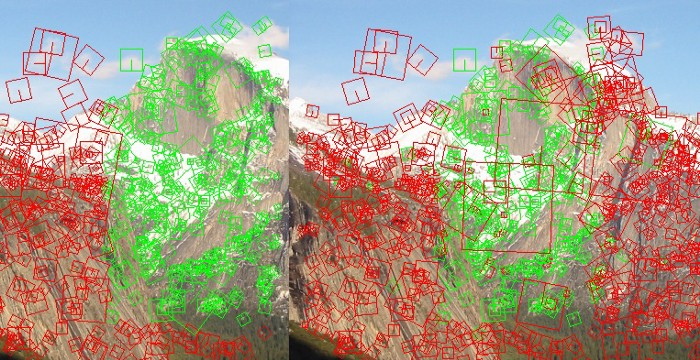# 所有特征提取与匹配算法通用过程

1. 找到那些周围有明显变化的像素点作为特征点。如下图所示，那些角点和边缘这些地方明显颜色变化的那些像素点被作为特征点。2. 提取这些特征点周围信息。一般是在当前这个点周围随机采样选几个像素点作为当前特征点的周围信息，或者画个圈圈进行采样。不同采样方法构成了不同算法。反正你想一个采样方法那你就创建了一种算法。

3. 特征点匹配。比如我要跟踪某个物体，我肯定是要先从这个物体提取一些特征点。然后看下一帧相同特征点的位置在哪，计算机就知道这个物体位置在哪了。怎么匹配？前面提到了我们第2步有提取当前特征点周围信息，只要周围信息一样那就是相同特征点。特征匹配也有很多种算法，最土的是前后两帧图片上的特征点一个一个的比对。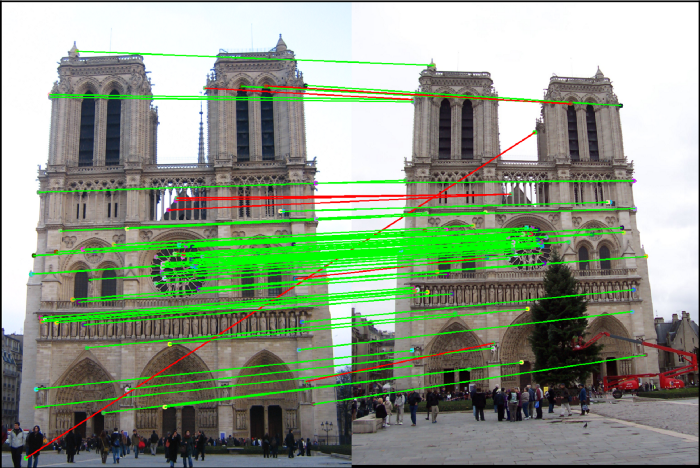记住学习任何特征提取与匹配算法都要时刻想起上面提到的三步骤。这样你不会太陷入那些书里面的细节中而学了很久都不懂。或者学完就忘。事实上那些算法非常简单，只不过你不知道他们各个步骤之间的联系是什么为什么这么设计。不知道这些当然就看不懂了。

# FAST特征点提取算法

FAST (Features from Accelerated Segment Test)是一个特征点提取算法的缩写。这是一个点提取算法。它原理非常简单，遍历所有的像素点，判断当前像素点是不是特征点的唯一标准就是在以当前像素点为圆心以3像素为半径画个圆（圆上有16个点），统计这16个点的像素值与圆心像素值相差比较大的点的个数。超过9个差异度很大的点那就认为圆心那个像素点是一个特征点。那么什么叫做差异度很大呢？答：就是像素值相减取绝对值，然后我们设置一个数字只要前面那个绝对值大于这个数字，那就认为差异大。比如我设置阈值是3。第1个像素点的像素值是4，中间圆心像素值是10，然后10-4=6，这是大于阈值3的。所以第1个像素点算所一个差异度较大的像素点。就这样**统计1~16个中有多少个是和圆心相比差异度比较大的点。只要超过9个那就认为圆心是一个特征点。**是不是很简单？其实这些算法只要你知道他们想干嘛，你也可以设计一个不错的算法的。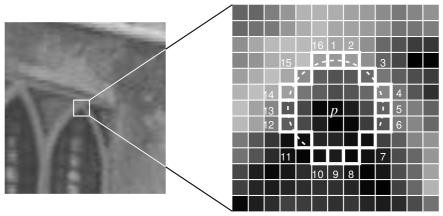为了简化问题我们构造一个带有角点的7x7的小图片，注意下面坐标轴单位是像素。（自己构造一些图片是检验我们实现的算法很重要的一个技能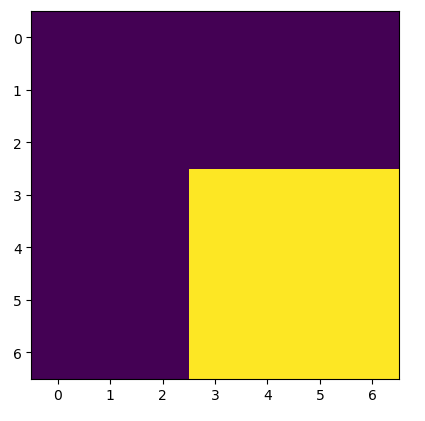然后使用bresenham算法画圆（如下图所示），可以看到周围有超过9个点与中心那个像素点的像素值很大差异。因此程序会判断当前圆心所在的像素点是关键点。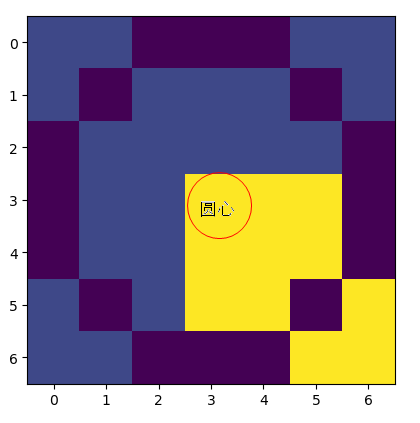现在稍微有一丝丝难度的是怎么画圆。因为这个圆它是一个像素一个像素的画。这个圆其实你自己可以随便设计一个算法画圆。今天我们要讲FAST算法当然还是介绍下他是怎么画圆的。他就用了最普通的图形学画圆算法（Bresenham 画圆法 ）。

其实到这里FAST算法我们就介绍完了。为了节省大家的时间（你的赞和关注是支持我分享的动力），我把Bresenham 画圆法也讲讲。

## Bresenham 布雷森汉姆算法画圆的原理与编程实现教程

注意：Bresenham的圆算法只是中点画圆算法的优化版本。区别在于Bresenham的算法只使用整数算术，而中点画圆法仍需要浮点数。你不了解中点画圆法并没有任何影响，因为他们只是思想一样，但是思路并不是一样。

Bresenham也是根据待选的两个点哪个离圆弧近就下一步选哪个。它是怎么判断的呢？这两个点一定有一个在圆弧内一个在圆弧外。到底选哪个？Bresenham的方法就是直接计算两个点离圆弧之间的距离，然后判断哪个更近就选哪个。如下图所示：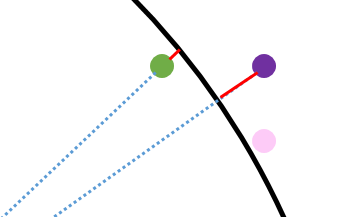那么怎么用数学量化他们离圆弧的距离呢？
答：前面我们提到了，当前粉红色这个点坐标为 ( x k , y k ) (x_k,y_k) ，下一步它有两种可能的走法绿色 ( x k − 1 , y k + 1 ) (x_k-1,y_k+1) ，紫色坐标为 ( x k , y k + 1 ) (x_k,y_k+1)
d 1 = ( x k − 1 ) 2 + ( y k + 1 ) 2 − r 2 d1 = (x_k-1)^2+(y_k+1)^2-r^2
d 2 = ( x k ) 2 + ( y k + 1 ) 2 − r 2 d2 = (x_k)^2+(y_k+1)^2-r^2
注意： d 1 = ( x k − 1 ) 2 + ( y k + 1 ) 2 − r 2 d1 = (x_k-1)^2+(y_k+1)^2-r^2 小于0的，因为绿色那个点一定在圆内侧。 d 2 = ( x k ) 2 + ( y k + 1 ) 2 − r 2 d2 = (x_k)^2+(y_k+1)^2-r^2 一定是大于等于0的，因为紫色那个点一定在圆外侧。

所以我们只用比较 P k = d 1 + d 2 P_k = d1+d2 到底是大于0还是小于0就能确定选哪个点了。大于0选绿色 ( x k − 1 , y k + 1 ) (x_k-1,y_k+1) 那个点（因为紫色那个点偏离圆弧程度更大）。小于0则选紫色 ( x k , y k + 1 ) (x_k,y_k+1) 那个点

好了Bresenham画圆法我讲完了

你或许会问，不对啊。我在网上看到的关于Bresenham画圆法的博客还有其他公式。确实我还有一个小细节没讲。你用上面的方法是已经可以画圆了，剩下的就是一些提高计算效率的小细节

P k = d 1 + d 2 = ( x k − 1 ) 2 + ( y k + 1 ) 2 − r 2 + ( x k ) 2 + ( y k + 1 ) 2 − r 2 P_k = d1+d2= (x_k-1)^2+(y_k+1)^2-r^2+(x_k)^2+(y_k+1)^2-r^2 这个公式走到下一步时候 P k + 1 = d 1 + d 2 P_{k+1} = d1+d2 又要重新计算。为了提高效率。人们就想能不能通过递推的方式来算 P k + 1 P_{k+1} ，即能不能找一个这样的公式 P k + 1 = P k + Z P_{k+1}=P_k+Z 提高计算效率。

这个也很简单，这个递推公式关键在于求Z。而我们变换下公式 P k + 1 = P k + Z P_{k+1}=P_k+Z 得到 Z = P k + 1 − P k Z=P_{k+1}-P_k
注意： P k = d 1 + d 2 = ( x k − 1 ) 2 + ( y k + 1 ) 2 − r 2 + ( x k ) 2 + ( y k + 1 ) 2 − r 2 P_k= d1+d2= (x_k-1)^2+(y_k+1)^2-r^2+(x_k)^2+(y_k+1)^2-r^2 我们已知的，而 P k + 1 P_{k+1} 这个根据 P k P_k 大于0还是小于0也可以算出来。

1. P k > = 0 P_k>=0 则证明靠近外侧的那个待选点 ( x k , y k + 1 ) (x_k,y_k+1) 离圆弧更远，所以我们下一步选的点是另外一个靠近内侧圆弧的那个点 ( x k − 1 , y k + 1 ) (x_k-1,y_k+1) 。也就是说第k+1步那个点 ( x k + 1 , y k + 1 ) = ( x k − 1 , y k + 1 ) (x_{k+1},y_{k+1})=(x_k-1,y_k+1)
Z = P k + 1 − P k = ( x k + 1 − 1 ) 2 + ( y k + 1 + 1 ) 2 − r 2 + ( x k + 1 ) 2 + ( y k + 1 + 1 ) 2 − r 2 − [ ( x k − 1 ) 2 + ( y k + 1 ) 2 − r 2 + ( x k ) 2 + ( y k + 1 ) 2 − r 2 ] = ( x k − 1 − 1 ) 2 + ( y k + 1 + 1 ) 2 − r 2 + ( x k − 1 ) 2 + ( y k + 1 + 1 ) 2 − r 2 − [ ( x k − 1 ) 2 + ( y k + 1 ) 2 − r 2 + ( x k ) 2 + ( y k + 1 ) 2 − r 2 ] = − 4 x k + 4 y k + 10 Z=P_{k+1}-P_k= (x_{k+1}-1)^2+(y_{k+1}+1)^2-r^2+(x_{k+1})^2+(y_{k+1}+1)^2-r^2 -[ (x_k-1)^2+(y_k+1)^2-r^2+(x_k)^2+(y_k+1)^2-r^2] \\ = (x_k-1-1)^2+(y_k+1+1)^2-r^2+(x_k-1)^2+(y_k+1+1)^2-r^2 -[ (x_k-1)^2+(y_k+1)^2-r^2+(x_k)^2+(y_k+1)^2-r^2]\\ =-4x_k+4y_k+10 。所以 P k + 1 = P k − 4 x k + 4 y k + 10 P_{k+1}=P_k-4x_k+4y_k+10
2. P k < 0 P_k<0 时，们下一步选的点是另外一个靠近内侧圆弧的那个点是 ( x k , y k + 1 ) (x_k,y_k+1) 。也就是说第k+1步那个点 ( x k + 1 , y k + 1 ) = ( x k , y k + 1 ) (x_{k+1},y_{k+1})=(x_k,y_k+1) 。我们看看现在的Z是多少。
Z = P k + 1 − P k = ( x k + 1 − 1 ) 2 + ( y k + 1 + 1 ) 2 − r 2 + ( x k + 1 ) 2 + ( y k + 1 + 1 ) 2 − r 2 − [ ( x k − 1 ) 2 + ( y k + 1 ) 2 − r 2 + ( x k ) 2 + ( y k + 1 ) 2 − r 2 ] = ( x k − 1 ) 2 + ( y k + 1 + 1 ) 2 − r 2 + ( x k ) 2 + ( y k + 1 + 1 ) 2 − r 2 − [ ( x k − 1 ) 2 + ( y k + 1 ) 2 − r 2 + ( x k ) 2 + ( y k + 1 ) 2 − r 2 ] = 4 y k + 6 Z=P_{k+1}-P_k= (x_{k+1}-1)^2+(y_{k+1}+1)^2-r^2+(x_{k+1})^2+(y_{k+1}+1)^2-r^2 -[ (x_k-1)^2+(y_k+1)^2-r^2+(x_k)^2+(y_k+1)^2-r^2] \\ = (x_k-1)^2+(y_k+1+1)^2-r^2+(x_k)^2+(y_k+1+1)^2-r^2 -[ (x_k-1)^2+(y_k+1)^2-r^2+(x_k)^2+(y_k+1)^2-r^2]\\ =4y_k+6 。所以 P k + 1 = P k + 4 y k + 6 P_{k+1}=P_k+4y_k+6
注意：根据初始点为(r,0)来计算 P k P_k 的初始值= − 2 r + 3 -2r+3
# -*- coding: utf-8 -*-
"""
Created on Sun Jul 21 15:02:36 2019
Bresenham画圆法实现
博客教程地址：
https://blog.csdn.net/varyshare/article/details/96724103
@author: 知乎@Ai酱
"""
import numpy as np
import matplotlib.pyplot as plt

img = np.zeros((105,105)) # 创建一个105x105的画布

def draw(x,y):
"""
绘制点(x,y)
注意：需要把(x,y)变换到数组坐标系（图形学坐标系）
因为数组(0,0)是左上，而原先坐标系(0,0)是中心点
而且数组行数向下是增加的。
"""
# 平移原点
x += int(img.shape/2)
y += int(img.shape/2)
#
img[-y,x] = 1
pass

r_pixel = 50 # 圆的半径,单位：像素
# 初始化,画第一个点，从水平最右边那个点开始画
(x,y) = (r_pixel,0)

"""
从定义来讲就是
P_k=d1+d2
d1 = 第1个下一步待选点离圆弧的距离（负数）
d2 = 第2个下一步待选点离圆弧的距离（正数）
但是为了提高效率通常使用递推来求P_{k+1}=P_k + 一个数
"""
P_k = -2*r_pixel + 3

# 迭代的求完1/8圆弧
while x>=y:
# 下一步有两个待选点，具体选哪个要看P_k>0 或 <0
if P_k>=0:# 外侧候选点偏离圆弧更远
P_k_next =  P_k - 4*x + 4*y + 10
(x_next,y_next) = (x-1, y+1)
else:# 内侧候选点偏离圆弧更远
P_k_next =  P_k + 4*y + 6
(x_next,y_next) = (x, y+1)
# 对称法画其他地方
draw(x,y)
draw(-x,y)
draw(x,-y)
draw(-x,-y)

draw(y,x)
draw(y,-x)
draw(-y,x)
draw(-y,-x)
# 更新坐标和P_k
(x,y) = (int(x_next),int(y_next))
P_k = P_k_next
pass
# 绘制图片
plt.imshow(img)


程序运行的结果为：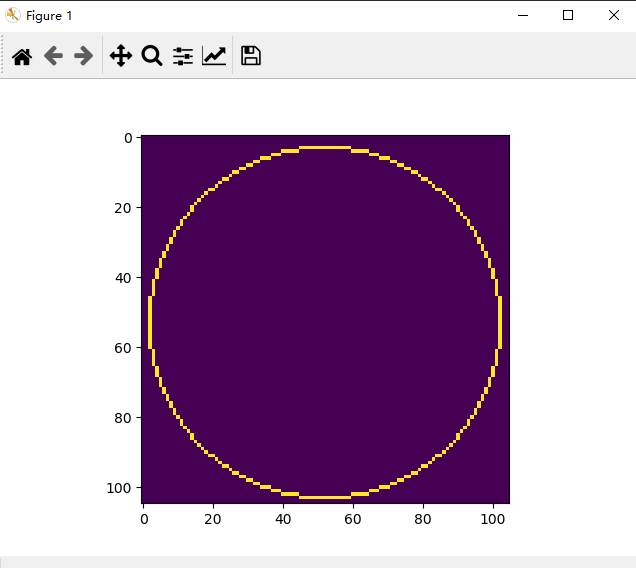上图的半径是40像素，当然FAST画圆的半径是3像素.我们只用修改一行设置半径的代码为r_pixel = 3 # 圆的半径,单位：像素。半径为3像素的圆如下图所示：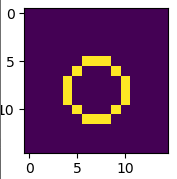使用OpenCV库中的FAST特征点检测算法

'''
Features from Accelerated Segment Test (FAST)
Python实现，
从0开始，最原始最简单的FAST特征点提取方法（无金字塔采样）
代码地址：https://github.com/varyshare/easy_slam_tutorial/tree/master/feature_extract
欢迎fork参与这个开源项目,star这项目
教程地址：https://blog.csdn.net/varyshare/article/details/96430924
代码没有怎么经过优化，所以会有0.8s左右的卡顿
'''
import numpy as np
import matplotlib.pyplot as plt
import cv2 # 用于读取图片

# 1. 读取图片(为了简化问题我就直接构造一个数组作为图片)

# 2. 设置参数
# 设置灰度值相差多大才算较大差异，
# 以及周围点超过多少个高差异点才算当前中心像素点是关键点
h_gray_scale = 20 # 在ORB特征提取中使用的FAST像素差阈值默认是20
k_diff_point = 9 # 超过k_diff_point个差异那就认为是关键点（周围共16个点）
r_pixel = 3 # 获取周围像素所用的圆的半径,单位：像素

# 3. 遍历所有的像素进行检测关键点
def bresenham_circle():
"""
return: 圆周上所有的点相对圆心的坐标列表。
即，返回圆心在(0,0)处时圆周上各点的坐标。
返回一个r_pixel*r_pixel的矩阵，圆周上的点标记为1，其他地方标记为0
"""

def save(x,y):
"""
把(x,y)加入到结果列表中
注意：需要把(x,y)变换到数组坐标系（图形学坐标系）
"""
pass

# 初始化,画第一个点，从水平最右边那个点开始画
(x,y) = (r_pixel,0)

"""
从定义来讲就是
P_k=d1+d2
d1 = 第1个下一步待选点离圆弧的距离（负数）
d2 = 第2个下一步待选点离圆弧的距离（正数）
但是为了提高效率通常使用递推来求P_{k+1}=P_k + 一个数
"""
P_k = -2*r_pixel + 3

# 迭代的求完1/8圆弧
while x>=y:
# 下一步有两个待选点，具体选哪个要看P_k>0 或 <0
if P_k>=0:# 外侧候选点偏离圆弧更远
P_k_next =  P_k - 4*x + 4*y + 10
(x_next,y_next) = (x-1, y+1)
else:# 内侧候选点偏离圆弧更远
P_k_next =  P_k + 4*y + 6
(x_next,y_next) = (x, y+1)
# 对称法画这对称的8个地方
save(x,y)
save(-x,y)
save(x,-y)
save(-x,-y)

save(y,x)
save(y,-x)
save(-y,x)
save(-y,-x)
# 更新当前坐标和P_k
(x,y) = (int(x_next),int(y_next))
P_k = P_k_next
pass

# 先bresenham算法算出半径为r_pixel时圆周上的点相对圆心的坐标
def key_point_test(_row,_col):
"""
检测像素点(_row,_col)是否是关键点。
满足关键点只有一个条件：周围16个像素点与中心像素点相比
差异度较大(>h_gray_scale)的像素点个数超过k_diff_point个
return: boolean
"""
# 获取以(_row,_col)为几何中心的7x7正方形区域内的像素值
surround_points = img[_row-3:_row+3+1,_col-3:_col+3+1]

# 获取圆周上的点与圆心的像素差值的绝对值
_dist = np.abs((surround_points - img[_row,_col])) * masked_canvas

if (_dist>h_gray_scale).sum()> k_diff_point:
return True
else:
return False

key_point_list = []

for row in range(r_pixel,img.shape-r_pixel):
for col in range(r_pixel,img.shape-r_pixel):

if key_point_test(row,col):
key_point_list.append(cv2.KeyPoint(x=row,y=col,_size=1))
else:
continue

pass
pass

img_with_keypoints = cv2.drawKeypoints(img,key_point_list,outImage=np.array([]),color=(0,0,255))
cv2.imshow("show key points",img_with_keypoints)
cv2.waitKey(0)


下图是我们对一个小图片进行检测程序运行的结果，可以看到最明显的几个角点找到了，但是也有几个点漏掉了。这是因为我们设置16个点中超过9个点和中心点不同这个数值9对于这张图来说大了些。你如果设置为7那就都能检测到了。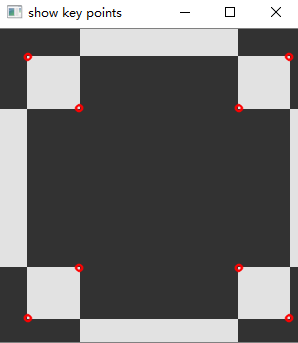参考文献：
 https://medium.com/software-incubator/introduction-to-feature-detection-and-matching-65e27179885d
 https://docs.opencv.org/3.0-beta/doc/py_tutorials/py_feature2d/py_fast/py_fast.html#fast
 https://medium.com/software-incubator/introduction-to-fast-features-from-accelerated-segment-test-4ed33dde6d65

展开全文• 使用OPENCV+VS2013实现SURF特征点提取，使用FLANN算法实现特征点匹配OPENCV
• 针对移动机器人导航过程中视觉图像处理速度慢以及特征点提取与匹配实时性和准确性差等特点，提出了一种基于SIFT特征提取算法KD树搜索匹配算法相结合的新方法，通过对候选特征点进行多次模糊处理，使其分布在高斯差...
• 本文总结了前人的研究成果，深入研究了各种特征提取特征点描述算法，在此基础上本文提出了一种新的图像匹配算法，运用角检测来实现图像特征提取，实现了基于图像匹配技术的实时电子稳像算法。
• 双目视觉是机器视觉中经典有效的方法。针对无色差光滑曲面特征点难以提取的问题, 提出一种用均匀...该算法直接对特征点进行提取匹配, 离散特征线获取特征点的方法相比精度更高, 可应用于曲面上的均匀抽样检测。
• 接下来，我们开始进入正题，特征点提取与匹配的实践操作。本篇文章中，我将着重介绍SIFT这种特征点以及做一个简单的特征点提取实践。 1.什么是特征点？ 首先我们来介绍一下什么是特征点，从字面的意思上来解释就是...
• 该文档介绍了基于Moravec算子的图像特征点提取以及基于相关系数法的图像匹配的基本原理编程实现方法，并附C#编写的程序源代码以及运行截图。
• 这篇论文主要是对FAST和BRISK做了改进 最后还对KAZE算法sift等进行比较和评估
•计算机视觉
• 特征提取的结果是把图像上的分为不同的子集，这些子集往往属于孤立的、连续的曲线或者连续的区域。 那么，什么样的才能称之为特征呢？ 至今为止特征并没有绝对精确的定义。特征的精确定义往往由问题或者应用...python 计算机视觉
• ## ORB特征提取与匹配

千次阅读 2018-04-09 10:07:37
ORB特征是目前最优秀的特征提取与匹配算法之一，下面具体讲解一下： 特征点的检测图像的特征点可以简单的理解为图像中比较显著显著的，如轮廓，较暗区域中的亮点，较亮区域中的暗等。ORB采用FAST（features ...
• 利用积分图像提出一种对光照变化鲁棒的快速关键点提取与匹配方法.首先,对基于黎曼积分的对比度拉伸响应,利用积分图像进行多尺度上采样滤波,快速提取光照鲁棒的局部特征,并在多分辨率框架下基于局部极大值检测多尺度...
• ## SIFT特征提取与匹配

千次阅读 2016-12-06 10:39:24
opencv下SIFT特征点提取与匹配SIFT：尺度不变特征转换，是一种电脑视觉的算法用来侦测描述影像中的局部特征。SIFT是基于图像外观的兴趣图像的大小旋转无关，对于噪声、光线、微观的视角容忍度也极高。SIFT...sift opencv...